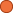• Managing your videos in Amara teams just got easier! Read about it in our latest blog post. Hide

## ← 03-06 Splay Tree

• 2 Followers
• 37 Lines

03-06 Splay Tree

### Get Embed Code x Embed video Use the following code to embed this video. See our usage guide for more details on embedding. Paste this in your document somewhere (closest to the closing body tag is preferable): ```<script type="text/javascript" src='https://amara.org/embedder-iframe'></script> ``` Paste this inside your HTML body, where you want to include the widget: ```<div class="amara-embed" data-url="http://www.youtube.com/watch?v=soybIVw_f_E" data-team="udacity"></div> ``` 1 Language

•English [en] incomplete

Showing Revision 2 created 07/16/2014 by Udacity Robot.

1. We'll that's enough abstract ideas for now without coverage.
2. So, let's take a concrete look of what a coverage can do for us in practice.
3. How it can help us do better testing.
4. What are we going to do here is look at some random open source Python codes
5. that implements a splay tree and before we go into the details
6. let me briefly explain what a splay tree is.
7. And broadly speaking a splay tree is just a kind of binary search tree.
8. And a binary search tree is a tree where every node has two leaves
9. and it supports operations such as insert, delete, and lookup.
10. The main important thing about a binary search tree,
11. the keys have to support an order in relation.
12. And so an order in relation is anything that assigns a total ordering to all the keys in the space.
13. Just a simple example if we're using integers for keys then we can use less than for order in relation.
14. Or for example, if we're using words as our keys, then we can use dictionary order.
15. And so again, it doesn't matter what kind of a data type the key is in a binary search tree.
16. All that really matters is the keys we're going to use to look up
17. elements in the tree have this order and relation.
18. The way the binary search tree is going to work is,
19. we're going to build up a tree under the invariant that the left child of any node
20. always has a key that's ordered before the key of the parent node
21. and the right child is always ordered after the parent node using the ordering.
22. And so, hopefully which we can see now,
23. is that if we build up some sort of a large tree with this kind of shape,
24. we have a procedure for fast lookup.
25. And the way that lookup works is, when we're searching for a particular key
26. in the binary search tree, we only have to walk one path from the root down to the leaves.
27. We always know which subtree might contain the key that we're looking for,
28. and of course, we have to actually go down into that subtree to see if it's there,
29. but the point is we only have to walk one path to the tree.
30. And the upside is that generally, operations on this kind of a search tree,
31. we're going to require a number of tree operations logarithmic in the number of tree nodes.
32. So for example, we if we have a tree with a million nodes and if that tree that we build ends
33. up being relatively balanced, then usually, we're going to expect to do about log base two
34. of a million tree operations as part of a lookup, or insert, or delete
35. and so we're going to end up doing roughly 20 operations.
36. So, you can see some speed number of operations is far lower than a number of nodes in a tree
37. that this is generally considered to be an efficient kind of a data structure.